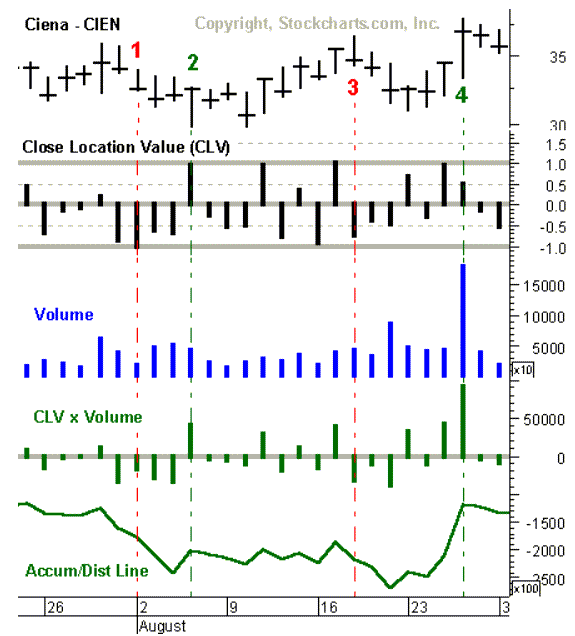## Accumulation/Distribution Line

The Accumulation/Distribution Line (A/D line) was developed by Marc Chaikin and is one of the popular volume flow indicators to assess the early cumulative flow of money into and out of a security, which can anticipate price moves of the stock.   An up-trending A/D Line suggests that buying pressure is building on higher volume, and a down-trending A/D Line indicates that selling pressure is building on higher volume. The basic premise behind the A/D line is that an increase in the volume of shares traded, e.g. per day, will precede an eventual move in the price of the stock.  Many times before a stock advances there will be a period of increased volume in the stock on the UP days just prior to the price move of the stock.  The A/D line focuses on the price action for a given period (e.g. daily) and generates a value based on the location of the close, relative to the range for the day. We will call this value the “Close Location Value” or CLV. The CLV ranges from plus one to minus one with the center point at zero.  Below is a summary of the the rules to calculate CLV.

1. If the stock closes on the high, the top of the range, then the value would be plus one.

2. If the stock closes above the midpoint of the high-low range, but below the high, then the value would be between zero and one.

3. If the stock closes exactly halfway between the high and the low, then the value would be zero.

4. If the stock closes below the midpoint of the high-low range, but above the low, then the value would be between zero and minus one.

5. If the stock closes on the low, the absolute bottom of the range, then the value would be minus one.

Once the CLV is calculated, it is then multiplied by the corresponding period’s volume, and the cumulative total forms the A/D Line.  Below is an example of CIEN and how the A/D line is calculated, courtesy of stockcharts.com.